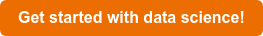# What size is this?

Suppose you want to predict what the length or width of a flower petal.
For this we can look for a relation between the two.

For this post we'll be looking at Linear Regression.

Linear regression is a test to see if two variables, let's say X and Y, are related so that when X increases; Y does as well. Y is therefor dependent on X, and if this relation is valid we can use a model to predict Y using X.

As a dataset we'll be using the iris dataset from sklearn.

We first import the dataset after which we'll take a look at the first few rows.

`iris = ds.load_iris() # the iris datasetdf = pd.DataFrame.from_records(data=iris.data, columns=iris.feature_names)df.head()`We want to find a relation between the width and length of a petal so we'll use them to plot a simple scatter plot.

`X = df['petal width (cm)']y = df['petal length (cm)']plt.scatter(X,y)plt.show()`Next we'll split the data, then train a linear model to see if the relation between the width and length is linear.

`# Split datasetX_train, X_test, y_train, y_test = train_test_split(X, y, test_size=0.3, random_state=0) # Train the model using the training setsregr.fit(X_train.values.reshape(-1, 1), y_train.values.reshape(-1, 1))`

`# predict lengthy_pred = regr.predict(X_test.values.reshape(-1, 1))`

After training our model and making a prediction between width and height we can plot both the test data and predicted results.

`plt.scatter(X_test,y_test,color='r')plt.scatter(X_test,y_pred,color='b', linewidth=2)plt.show()`Looking at the plot we can visually determine there are no clear outliers to the data which means a linear relationship between petal width and length is valid.

Using linear regression we can determine linear relationships between data, in this example; the relation between a flower petal's width and length.

Get the code here!